## Thursday, May 30, 2013

### If…then in Japan

If Japan starts to spiral out of control, then what do they do? A spiral would be a sudden move higher in JGB rates with a simultaneous crash in the Japanese Yen. Their response would be to try to slow the positive feedback loop through external intervention. Fortunately for Japan, they have amassed a significant reserve position of 1.2 trillion compared to a US reserve position of 135 billion, so they do have some firepower.
``````require(latticeExtra)
require(quantmod)

japanReserves <- getSymbols("TRESEGJPM052N", src = "FRED",
auto.assign = FALSE)
asTheEconomist(xyplot(japanReserves, scales = list(y = list(rot = 1)),
main = "Japan Foreign Reserves excluding Gold"))``````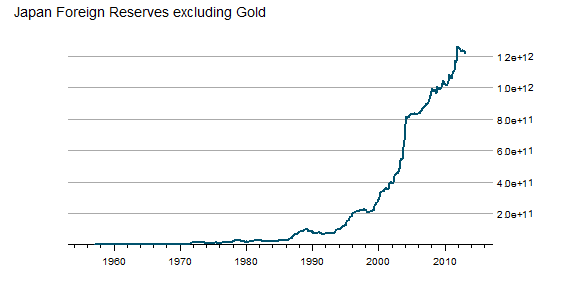From TimelyPortfolio

Based on data from the US Treasury, 1.1 trillion of the 1.2 trillion of Japanese foreign reserves are US Treasury bonds. If Japan decides (is forced) to liquidate, the only thing they have to liquidate are US Treasury bonds. Who will buy if they sell? What happens next?
``````# get data from US Treasury for top 10 foreign
# holders of US treasuries
skip = 4, nrows = 13, stringsAsFactors = FALSE)
top10 <- data.frame(foreignUSTreas[4:13, 1:2])
colnames(top10) <- c("Country", "Value")
top10\$Value <- as.numeric(top10\$Value)
top10\$Country <- factor(top10\$Country, levels = top10\$Country[order(top10\$Value)])

barchart(Country ~ Value, data = top10, origin = 0,
xlab = "Value (in \$Billions)", main = "Foreign Holders of US Treasuries - Top 10",
scales = list(y = list(alternating = 3)), par.settings = theEconomist.theme(box = "transparent"),
lattice.options = theEconomist.opts())``````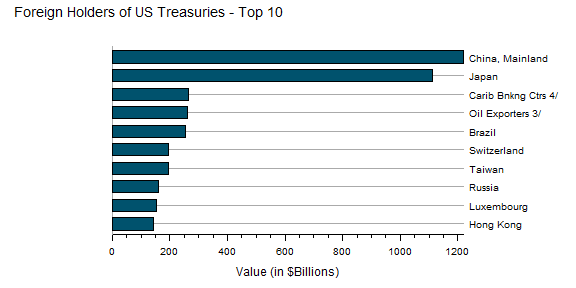From TimelyPortfolio

For those comparing the Federal Reserve H4 data to the US Treasury TIC data, this is a very good summary http://www.treasury.gov/resource-center/data-chart-center/tic/Pages/ticfaq2.aspx#q10.

## Tuesday, May 28, 2013

### Intended or Unintended Consequences

A quick glimpse at the US 10y Treasury Bond rate since 2000 seems benign with low volatility and a general downward trend.

``require(latticeExtra)require(quantmod)US10y <- getSymbols("^TNX", from = "2000-01-01", auto.assign = FALSE)[,     4]asTheEconomist(xyplot(US10y, scales = list(y = list(rot = 1)),     main = "US 10y Yield Since 2000"))``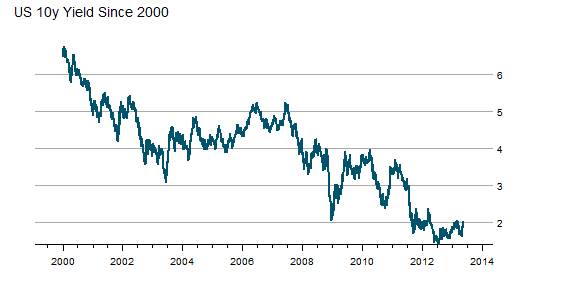From TimelyPortfolio

However, if we plot the change in rate over 252 days or 1 year, we might wonder whether the rate is as benign as we initially surmised. Although this might seem like an acceptable intended consequence of the aggressive monetary easing by the US Fed, I fear that we will face the historically normal unintended consequences.

``p1 <- asTheEconomist(xyplot(US10y - lag(US10y, 252),     scales = list(y = list(rot = 0)), main = "US 10y Yield - Yearly Change"))p1``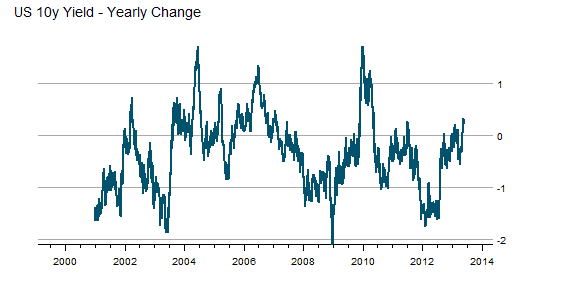From TimelyPortfolio

And if we use some Vanguard US Bond Funds (vbmfx and vustx) as a proxy for the US bond market Approaching the Zero Bound - Bonds, we see that we are now in a market different than the last 4 years.

``require(directlabels)require(reshape2)getSymbols("VUSTX", from = "1990-01-01")``
``##  "VUSTX"``
``getSymbols("VBMFX", from = "1990-01-01")``
``##  "VBMFX"``
``bonds.tr <- merge(ROC(VUSTX[, 6], 250), ROC(VBMFX[,     6], 250))colnames(bonds.tr) <- c("VanguardLongTsy", "VanguardTotBnd")bond.funds.melt <- melt(as.data.frame(cbind(as.Date(index(bonds.tr)),     coredata(bonds.tr))), id.vars = 1)colnames(bond.funds.melt) <- c("date", "fund", "totret250")bond.funds.melt\$date <- as.Date(bond.funds.melt\$date)p2 <- direct.label(xyplot(bonds.tr, screens = 1, ylim = c(-0.35,     0.35), scales = list(y = list(rot = 0)), col = theEconomist.theme()\$superpose.line\$col,     par.settings = theEconomist.theme(box = "transparent"),     lattice.options = theEconomist.opts(), xlab = NULL,     main = "Vanguard Bond Funds 250 Day Total Return"),     list("last.points", hjust = 1, cex = 1.2))p2``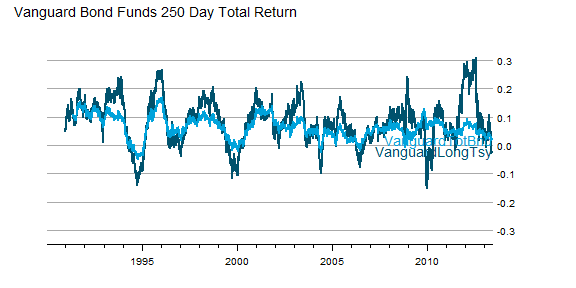From TimelyPortfolio
``p3 <- asTheEconomist(horizonplot(totret250 ~ date |     fund, origin = 0, horizonscale = 0.05, data = bond.funds.melt,     strip = TRUE, strip.left = FALSE, par.strip.text = list(cex = 1.1),     layout = c(1, 2), main = "Vanguard Bond Funds 250 Day Total Return"))p3``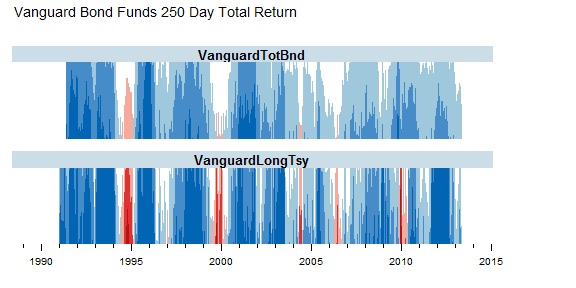From TimelyPortfolio
``# print(p2,position=c(0,0.4,1,1),more=TRUE)# print(update(p3,main=NULL),position=c(0,0,1,0.5))``

### Comparison to Japan

For some reference, let's look at a country getting quite a bit of attention lately in the press. We can add US yields to my favorite chart from Japan - JGB Yields–More Lattice Charts. Most of the difference is in the short end of the curve where the US is still near the minimum since 2012 while Japan is near the max out to 7 years.

``url <- "http://www.mof.go.jp/english/jgbs/reference/interest_rate/"filenames <- paste("jgbcme",c("","_2010","_2000-2009","_1990-1999","_1980-1989","_1974-1979"),".csv",sep="")#load all data and combine into one jgb data.framejgb <- read.csv(paste(url,filenames,sep=""),stringsAsFactors=FALSE)for (i in 2:length(filenames)) {  jgb <- rbind(jgb,read.csv(paste(url,"/historical/",filenames[i],sep=""),stringsAsFactors=FALSE))}#now clean up the jgb data.frame to make a jgb xtsjgb.xts <- as.xts(data.matrix(jgb[,2:NCOL(jgb)]),order.by=as.Date(jgb[,1]))colnames(jgb.xts) <- paste0(gsub("X","JGB",colnames(jgb.xts)))#get Yen from the Fed#getSymbols("DEXJPUS",src="FRED")xtsMelt <- function(data) {  require(reshape2)  #translate xts to time series to json with date and data  #for this behavior will be more generic than the original  #data will not be transformed, so template.rmd will be changed to reflect  #convert to data frame  data.df <- data.frame(cbind(format(index(data),"%Y-%m-%d"),coredata(data)))  colnames(data.df) = "date"  data.melt <- melt(data.df,id.vars=1,stringsAsFactors=FALSE)  colnames(data.melt) <- c("date","indexname","value")  #remove periods from indexnames to prevent javascript confusion  #these . usually come from spaces in the colnames when melted  data.melt[,"indexname"] <- apply(matrix(data.melt[,"indexname"]),2,gsub,pattern="[.]",replacement="")  return(data.melt)  #return(df2json(na.omit(data.melt)))}jgb.melt <- xtsMelt(jgb.xts["2012::",])jgb.melt\$date <- as.Date(jgb.melt\$date)jgb.melt\$value <- as.numeric(jgb.melt\$value)jgb.melt\$indexname <- factor(  jgb.melt\$indexname,  levels = colnames(jgb.xts))jgb.melt\$maturity <- as.numeric(  substr(    jgb.melt\$indexname,    4,    length( jgb.melt\$indexname ) - 4  ))jgb.melt\$country <- rep( "Japan", nrow( jgb.melt ))#now get the US bonds from FREDUSbondssymbols <- paste0("DGS",c(1,2,3,5,7,10,20,30))ust.xts <- xts()for (i in 1:length( USbondssymbols ) ) {  ust.xts <- merge(     ust.xts,    getSymbols(      USbondssymbols[i],     auto.assign = FALSE,     src = "FRED"    )  )}ust.melt <- na.omit( xtsMelt( ust.xts["2012::",] ) )ust.melt\$date <- as.Date(ust.melt\$date)ust.melt\$value <- as.numeric(ust.melt\$value)ust.melt\$indexname <- factor(  ust.melt\$indexname,  levels = colnames(ust.xts))ust.melt\$maturity <- as.numeric(  substr(    ust.melt\$indexname,    4,    length( ust.melt\$indexname ) - 4  ))ust.melt\$country <- rep( "US", nrow( ust.melt ))bonds.melt <- rbind( jgb.melt, ust.melt )p4 <- xyplot(  value ~ date | maturity,  groups = country,  data = bonds.melt,  type = "l",  col = c(brewer.pal(9,"Blues"),brewer.pal(9,"Blues")),  layout = c( length( unique( bonds.melt\$maturity ) ), 1 ),  panel = function(x, y, subscripts, col, ...) {    panel.abline(      h = c(         sapply( by( y, bonds.melt[subscripts,"country"], summary ), min ),        sapply( by( y, bonds.melt[subscripts,"country"], summary ), max )      ),      #if(!col){      col = col[1:length(unique(bonds.melt[subscripts,"country"]))]      #} else col = trellis.par.get()\$superpose.line\$col[1:length(unique(bonds.melt[subscripts,"country"]))]    )    panel.xyplot( x = x, y = y, subscripts = subscripts, col = col, ... )    panel.text(      x = x[ length(unique(x)) / 2],      y = mean(        c(          sapply( by( y, bonds.melt[subscripts,"country"], summary ), max ),          sapply( by( y, bonds.melt[subscripts,"country"], summary ), min )        )      ),      labels = paste0(unique(bonds.melt\$maturity)[panel.number()],"y"),      cex = 0.8,      #font = 2,      col = "black",      adj = c(0.5,0.5)    )  },  scales = list(     x = list(      draw = FALSE      #tck = c(1,0),      #alternating = 1,      #at = min(bonds.melt\$date),      #labels = format(min(bonds.melt\$date),"%Y")    ),    y = list( tck = c(1,0), lwd = c(0,1) )  ),  strip = FALSE,  par.settings = list(axis.line = list(col = 0)),  xlab = NULL,  ylab = "Yield",  main = "JGB and US Yields by Maturity Since Jan 2012")p4 <- p4 + layer(  panel.abline(    h = pretty(bonds.melt\$value,4),    lty = 3  ))p4``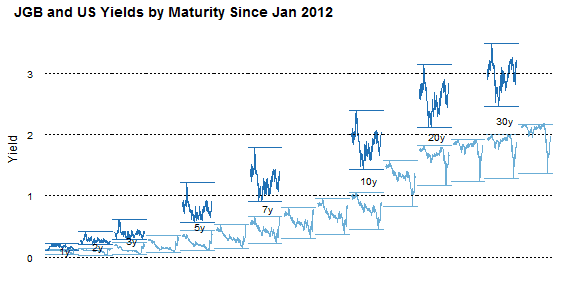From TimelyPortfolio

### Replicate

Gist source: https://gist.github.com/timelyportfolio/5665790

## Wednesday, May 15, 2013

### Even More JGB Yield Charts with R lattice

See the last post for all the details. I just could not help creating a couple more.

### Variations on Favorite Plot - Time Series Line of JGB Yields by Maturity

``p2 <- xyplot(value ~ date | indexname, data = jgb.melt,     type = "l", layout = c(length(unique(jgb.melt\$indexname)),         1), panel = function(x, y, ...) {        panel.abline(h = c(min(y), max(y)))        panel.xyplot(x = x, y = y, ...)        panel.text(x = x[length(x)/2], y = max(y),             labels = levels(jgb.melt\$indexname)[panel.number()],             cex = 0.7, pos = 3)    }, scales = list(x = list(tck = c(1, 0), alternating = 1),         y = list(tck = c(1, 0), lwd = c(0, 1))), strip = FALSE,     par.settings = list(axis.line = list(col = 0)),     xlab = NULL, ylab = "Yield", main = "JGB Yields by Maturity Since Jan 2012")p2 <- p2 + layer(panel.abline(h = pretty(jgb.melt\$value),     lty = 3))p2``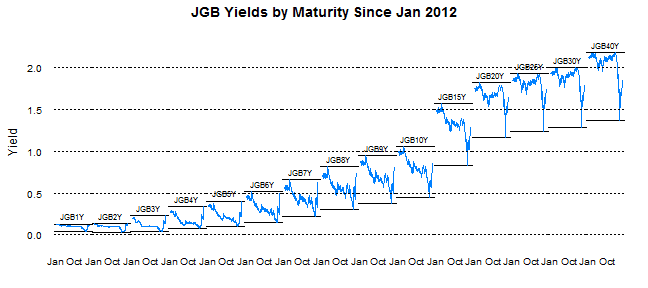From TimelyPortfolio

``jgb.xts.diff <- jgb.xts["2012::", ] - matrix(rep(jgb.xts["2012::",     ][1, ], NROW(jgb.xts["2012::", ])), ncol = NCOL(jgb.xts),     byrow = TRUE)jgb.diff.melt <- xtsMelt(jgb.xts.diff)jgb.diff.melt\$date <- as.Date(jgb.diff.melt\$date)jgb.diff.melt\$value <- as.numeric(jgb.diff.melt\$value)jgb.diff.melt\$indexname <- factor(jgb.diff.melt\$indexname,     levels = colnames(jgb.xts))p4 <- xyplot(value ~ date | indexname, data = jgb.diff.melt,     type = "h")update(p2, ylim = c(min(jgb.diff.melt\$value), max(jgb.melt\$value) +     0.5)) + p4``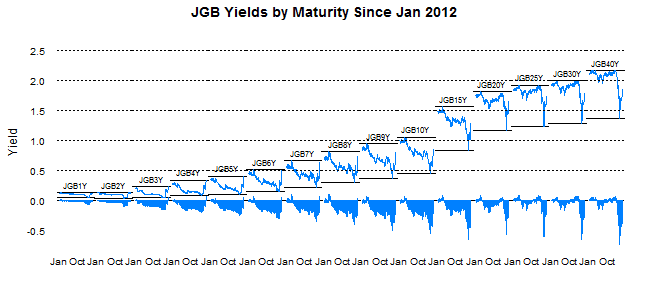From TimelyPortfolio

``update(p2, ylim = c(min(jgb.diff.melt\$value), max(jgb.melt\$value) +     0.5), par.settings = list(axis.line = list(col = "gray70"))) +     update(p4, panel = function(x, y, col, ...) {        # do color scale from red(negative) to        # blue(positive)        cc.palette <- colorRampPalette(c(brewer.pal("Reds",             n = 9), "white", brewer.pal("Blues",             n = 9)))        cc.levpalette <- cc.palette(20)        cc.levels <- level.colors(y, at = do.breaks(c(-0.3,             0.3), 20), col.regions = cc.levpalette)        panel.xyplot(x = x, y = y, col = cc.levels,             ...)    })``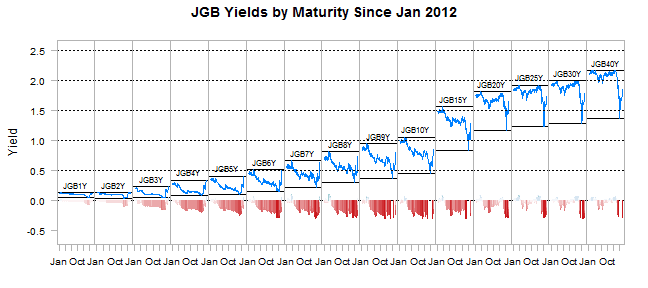From TimelyPortfolio

``p5 <- horizonplot(value ~ date | indexname, data = jgb.diff.melt,     layout = c(1, length(unique(jgb.diff.melt\$indexname))),     scales = list(x = list(tck = c(1, 0))), xlab = NULL,     ylab = NULL)p5``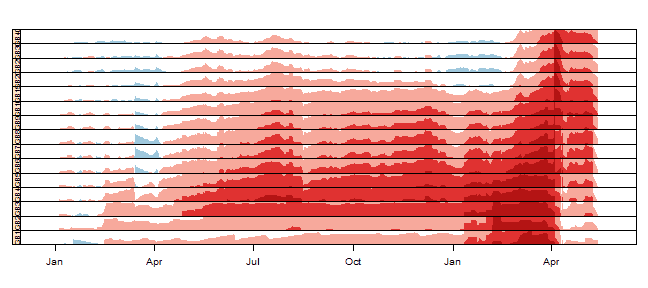From TimelyPortfolio

``update(p2, ylim = c(0, max(jgb.melt\$value) + 0.5),     panel = panel.xyplot) + p5 + update(p2, ylim = c(0,     max(jgb.melt\$value)))``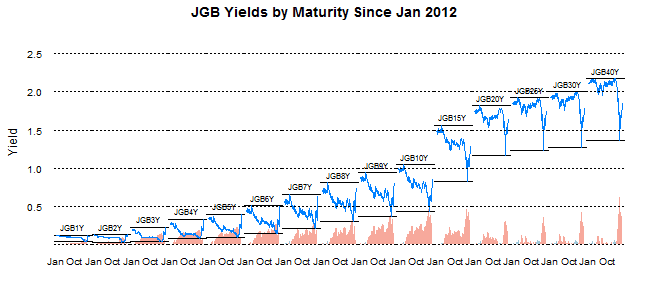From TimelyPortfolio

### Variations on Yield Curve Evolution with Opacity Color Scale

``# add alpha to colorsaddalpha <- function(alpha = 180, cols) {    rgbcomp <- col2rgb(cols)    rgbcomp <- alpha    return(rgb(rgbcomp, rgbcomp, rgbcomp,         rgbcomp, maxColorValue = 255))}p3 <- xyplot(value ~ indexname, group = date, data = jgb.melt,     type = "l", lwd = 2, col = sapply(400/(as.numeric(Sys.Date() -         jgb.melt\$date) + 1), FUN = addalpha, cols = brewer.pal("Blues",         n = 9)), main = "JGB Yield Curve Evolution Since Jan 2012")p3 <- update(asTheEconomist(p3), scales = list(x = list(cex = 0.7))) +     layer(panel.text(x = length(levels(jgb.melt\$indexname)),         y = 0.15, label = "source: Japanese Ministry of Finance",         col = "gray70", font = 3, cex = 0.8, adj = 1))# make point rather than lineupdate(p3, type = "p")``From TimelyPortfolio

``# make point with just most current curve as lineupdate(p3, type = "p") + xyplot(value ~ indexname,     data = jgb.melt[which(jgb.melt\$date == max(jgb.melt\$date)),         ], type = "l", col = brewer.pal("Blues", n = 9))``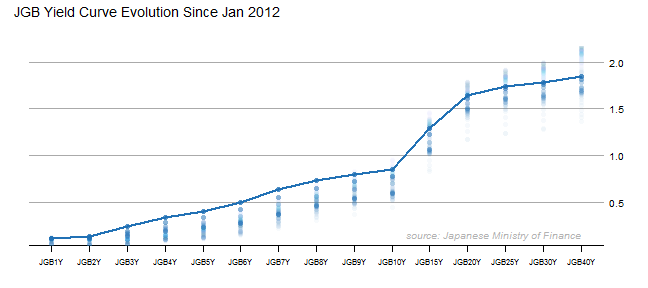From TimelyPortfolio

### Japan - JGB Yields–More Lattice Charts

This blog is littered with posts about Japan. In one sentence, I think Japan presents opportunity and is a very interesting real-time test of much of my macro thinking. Proper visualization is absolutely essential for me to understand all of the dynamics. The R packages lattice and the new rCharts give me the power to see. I thought some of my recent lattice charts might help or interest some folks.

### Get and Transform the Data

``# get Japan yield data from the Ministry of# Finance Japan data goes back to 1974require(xts)# require(clickme)require(latticeExtra)url <- "http://www.mof.go.jp/english/jgbs/reference/interest_rate/"filenames <- paste("jgbcme", c("", "_2010", "_2000-2009",     "_1990-1999", "_1980-1989", "_1974-1979"), ".csv",     sep = "")# load all data and combine into one jgb# data.framejgb <- read.csv(paste(url, filenames, sep = ""),     stringsAsFactors = FALSE)for (i in 2:length(filenames)) {    jgb <- rbind(jgb, read.csv(paste(url, "/historical/",         filenames[i], sep = ""), stringsAsFactors = FALSE))}# now clean up the jgb data.frame to make a jgb# xtsjgb.xts <- as.xts(data.matrix(jgb[, 2:NCOL(jgb)]),     order.by = as.Date(jgb[, 1]))colnames(jgb.xts) <- paste0(gsub("X", "JGB", colnames(jgb.xts)),     "Y")# get Yen from the Fed# getSymbols('DEXJPUS',src='FRED')xtsMelt <- function(data) {    require(reshape2)    # translate xts to time series to json with date    # and data for this behavior will be more generic    # than the original data will not be transformed,    # so template.rmd will be changed to reflect    # convert to data frame    data.df <- data.frame(cbind(format(index(data),         "%Y-%m-%d"), coredata(data)))    colnames(data.df) = "date"    data.melt <- melt(data.df, id.vars = 1, stringsAsFactors = FALSE)    colnames(data.melt) <- c("date", "indexname", "value")    # remove periods from indexnames to prevent    # javascript confusion these . usually come from    # spaces in the colnames when melted    data.melt[, "indexname"] <- apply(matrix(data.melt[,         "indexname"]), 2, gsub, pattern = "[.]", replacement = "")    return(data.melt)    # return(df2json(na.omit(data.melt)))}jgb.melt <- xtsMelt(jgb.xts["2012::", ])jgb.melt\$date <- as.Date(jgb.melt\$date)jgb.melt\$value <- as.numeric(jgb.melt\$value)jgb.melt\$indexname <- factor(jgb.melt\$indexname, levels = colnames(jgb.xts))``

### Favorite Plot - Time Series Line of JGB Yields by Maturity

``p2 <- xyplot(value ~ date | indexname, data = jgb.melt,     type = "l", layout = c(length(unique(jgb.melt\$indexname)),         1), panel = function(x, y, ...) {        panel.abline(h = c(min(y), max(y)))        panel.xyplot(x = x, y = y, ...)        panel.text(x = x[length(x)/2], y = max(y),             labels = levels(jgb.melt\$indexname)[panel.number()],             cex = 0.7, pos = 3)    }, scales = list(x = list(tck = c(1, 0), alternating = 1),         y = list(tck = c(1, 0), lwd = c(0, 1))), strip = FALSE,     par.settings = list(axis.line = list(col = 0)),     xlab = NULL, ylab = "Yield", main = "JGB Yields by Maturity Since Jan 2012")p2 + layer(panel.abline(h = pretty(jgb.melt\$value),     lty = 3))``From TimelyPortfolio

### Good Chart but Not a Favorite

As you can tell, I did not spend a lot of time formatting this one.

``p1 <- xyplot(value ~ date | indexname, data = jgb.melt,     type = "l")p1``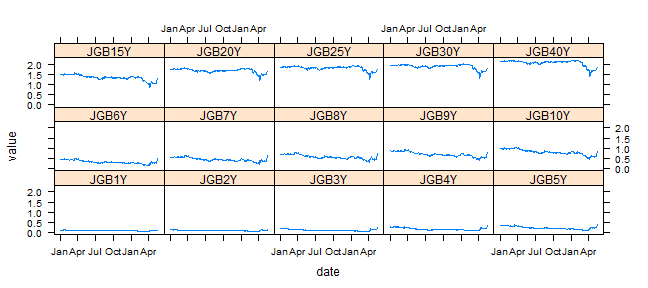From TimelyPortfolio

### Another Favorite - Yield Curve Evolution with Opacity Color Scale

``# add alpha to colorsaddalpha <- function(alpha = 180, cols) {    rgbcomp <- col2rgb(cols)    rgbcomp <- alpha    return(rgb(rgbcomp, rgbcomp, rgbcomp,         rgbcomp, maxColorValue = 255))}p3 <- xyplot(value ~ indexname, group = date, data = jgb.melt,     type = "l", lwd = 2, col = sapply(255/(as.numeric(Sys.Date() -         jgb.melt\$date) + 1), FUN = addalpha, cols = brewer.pal("Blues",         n = 9)), main = "JGB Yield Curve Evolution Since Jan 2012")update(asTheEconomist(p3), scales = list(x = list(cex = 0.7))) +     layer(panel.text(x = length(levels(jgb.melt\$indexname)),         y = 0.15, label = "source: Japanese Ministry of Finance",         col = "gray70", font = 3, cex = 0.8, adj = 1))``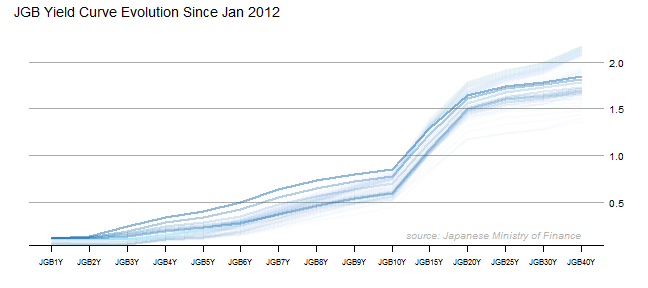From TimelyPortfolio

code at Gist

## Friday, May 3, 2013

### Old Post with New d3 Life–GARCH and MA Performance

Parallel coordinates become much more useful when they are interactive, so I recreated one of my favorite blog posts "Trend is Not Your Friend" Applied to 48 Industries and convert the chart to a living breathing d3 parallel coordinates chart courtesy of Ramnath Vaidyanathan's rCharts and Kai Chang's d3.parcoords. I will convert the scatter and horizon plots in a later post.  See here for the finished product.  Here or embedded below is the quick Youtube video showing the creation process.

## Thursday, May 2, 2013

### Changing The Presidential Election with R in the Browser

After I finished with the tutorial post d3 <- R with rCharts and slidify and then saw R creates d3/javascript charts in Ipython Style Notebook, a light clicked.  I could finally answer the lingering question I have had ever since I saw the NYT 512 Path to the White House brilliantly created by Mike Bostock and Shan Carter with d3.

What would happen if different states had a different number of votes?

I never dreamed that I would be able to answer my own question by live R coding in the browser in an Ipython style notebook.  I felt like I needed a screencast to prove it.  Here is the short version.

Here is the extended version with director commentary (in type).

Thanks Ramnath Vaidyanathan, Yihui Xie, and Mike Bostock.

## Wednesday, May 1, 2013

### R creates d3/javascript charts in Ipython Style Notebook

I am not sure I have ever done a post like this, but I was so blown away I had to do this post simply to embed this amazing Youtube video from the author of the R packages rCharts and slidify.  Watch this screencast as he creates d3/raphael charts from R using rCharts integration with polycharts, nvd3, xCharts, and morris.  To top it off, he does it all using shiny to create an Ipython type working environment.